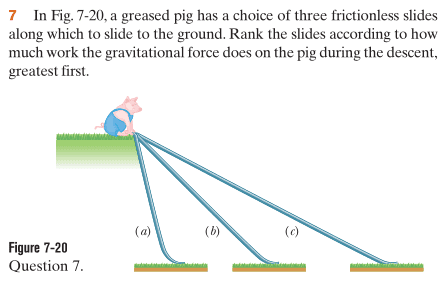# Confused with inclined plane and work done by gravity

## Homework Statement## Homework Equations

W_g = mgdcos(phi)

## The Attempt at a Solution

So the angle between the force doing work (x component of F_g) and the displacement (down the hill) is 0 degrees. aka phi is 0 degrees. This is true for (a), (b) and (c).

so since cos(0) = 1, the work done by gravity is just mgd. d is greatest for (c), then (b) then (a), so W_g is greatest for (c) then (b) then (a)

However the answers say that work done by gravity is same in all cases.

The textbook says work is generally path dependent, however when only conservative forces (like gravity) are acting, work is not path dependent. So I have a flaw in my understanding somewhere.

potential gravitational energy = mgh, and height is the same in all cases. Can someone explain how this all works?

DrClaude
Mentor
So the angle between the force doing work (x component of F_g) and the displacement (down the hill) is 0 degrees. aka phi is 0 degrees. This is true for (a), (b) and (c).
This is not correct. Consider when the pig is midway along slide (c). In what direction is Fg pointing? In what direction is the displacement d?

This is not correct. Consider when the pig is midway along slide (c). In what direction is Fg pointing? In what direction is the displacement d?

F_g is straight down (x component is still down the hill) and displacement is up the ramp? So the angle isn't 0 degrees anymore?

DrClaude
Mentor
F_g is straight down (x component is still down the hill) and displacement is up the ramp?
Not exactly "up" the ramp, belong along it.

So the angle isn't 0 degrees anymore?
Yes. To find the total work done by gravity, you would have to integrate over the entire path, with the angle φ not constant but varying with position depending on the exact shape of the slide.

Last edited:
ehild
Homework Helper
Not exactly "up" the ramp, belong along it.

Yes. To find the total work, you would have to integrate over the entire path, with the angle φ not constant but varying with position depending on the exact shape of the slide.
Not the total work was asked but the work of gravity. What do you think about the gravitational force? Is it conservative?

ehild
Homework Helper

## Homework Statement## Homework Equations

W_g = mgdcos(phi)

## The Attempt at a Solution

So the angle between the force doing work (x component of F_g) and the displacement (down the hill) is 0 degrees.
You should know the meaning of the notations you use. So what is phi in the formula for work?

DrClaude
Mentor
Not the total work was asked but the work of gravity.Yes, that's what I meant. I'll correct my post.

ehild
Homework HelperYes, that's what I meant. I'll correct my post.
Is the force of gravity conservative? What do you know about the work done by a conservative force?